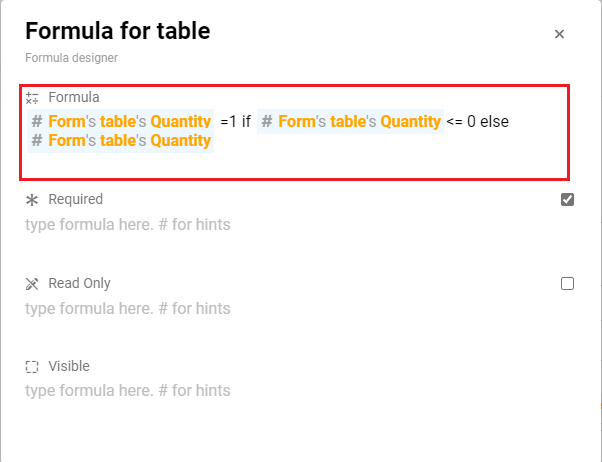# Set a default values when we create a new row

To setting a default value to an information when we creating a new row, we can done that with using the if condition in the formula.

First, in to the Studio go to the table where I would like to add the default value for it`s new row. Select the formula icon.

Next, By using the If else … condition we can make it execute the column “Quantity”= 1 when the column “Quantity” is less than(<=) 1, there is because the default value when we added a new row is 0 for an integer information. So, after this we need to add the else is the column “Quantity”. If not, the column value will always become 0 or None.After this configuration, the result will become as below:

1. Create a new row at the table

2. The column “Quantity” will be created with the value “1” as default instead of “0”

2 Likes